### Home > AC > Chapter 9 > Lesson 9.1.2 > Problem9-20

9-20.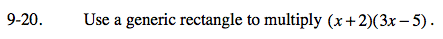Multiply each column value by each row value to fill the rectangle.

= 3x² + x − 10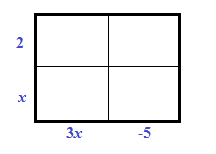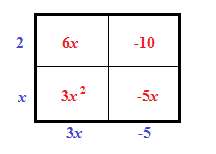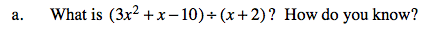3x² + x−10 is the same as (x + 2)(3x − 5).

The product divided by one factor is the other factor.

3x − 5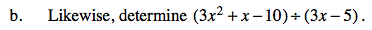See part (a).